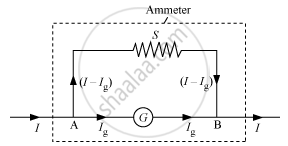Department of Pre-University Education, KarnatakaPUC Karnataka Science Class 12

# Can a galvanometer as such be used for measuring the current? Explain. - Physics

Can a galvanometer as such be used for measuring the current? Explain.

#### Solution

Yes a galvanometer can be used for measuring the current

Conversion of a galvanometer into an ammeterA shunt (low resistance) is connected in parallel with a  galvanometer.

S=(I_g/(I-I_g))G

Here,

I = total current in circuit

G = resistance of the galvanometer

S = resistance of the shunt

Ig = current through the galvanometer

Concept: Moving Coil Galvanometer
Is there an error in this question or solution?